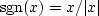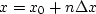# RATional FORtran == Ratfor

Bare-bones Fortran is our most universal computer language for computational physics. For general programming, however, it has been surpassed by C. ``Ratfor" is Fortran with C-like syntax. I believe Ratfor is the best available expository language for mathematical algorithms. Ratfor was invented by the people who invented C. Ratfor programs are converted to Fortran with the Ratfor preprocessor. Since the preprocessor is publicly available, Ratfor is practically as universal as Fortran.You will not really need the Ratfor preprocessor or any precise definitions if you already know Fortran or almost any other computer language, because then the Ratfor language will be easy to understand. Statements on a line may be separated by ``;." Statements may be grouped together with braces { }. Do loops do not require statement numbers because { } defines the range. Given that if( ) is true, the statements in the following { } are done. else{ } does what you expect. We may not contract else if to elseif. We may always omit the braces { } when they contain only one statement. break will cause premature termination of the enclosing { }. break 2 escapes from {{ }}. while( ) { } repeats the statements in { } while the condition ( ) is true. repeat { ... } until( ) is a loop that tests at the bottom. A looping statement more general than do is for(initialize; condition; reinitialize) { }. next causes skipping to the end of any loop and a retrial of the test condition. next is rarely used, but when it is, we must beware of an inconsistancy between Fortran and C-language. Where Ratfor uses next, the C-language uses continue (which in Ratfor and Fortran is merely a place holder for labels). The Fortran relational operators .gt., .ge., .ne., etc. may be written >, >=, !=, etc. The logical operators .and. and .or. may be written && and ||. Anything from a # to the end of the line is a comment. Anything that does not make sense to the Ratfor preprocessor, such as Fortran input-output, is passed through without change. (Ratfor has a switch statement but we never use it because it conflicts with the implicit undefined declaration. Anybody want to help us fix the switch in public domain ratfor?)

Indentation in Ratfor is used for readability. It is not part of the Ratfor language. Choose your own style. I have overcondensed. There are two pitfalls associated with indentation. The beginner's pitfall is to assume that a do loop ends where the indentation ends. The loop ends after the first statement. A larger scope for the do loop is made by enclosing multiple statements in braces. The other pitfall arises in any construction like if() ... if() ... else. The else goes with the last if() regardless of indentation. If you want the else with the earlier if(), you must use braces like if() { if() ... } else ....

The most serious limitation of Fortran-77 is its lack of ability to allocate temporary memory. I have written a preprocessor to Ratfor or Fortran to overcome this memory-allocation limitation. This program, named sat,  allows subroutines to include the declaration temporary real data(n1,n2), so that memory is allocated during execution of the subroutine where the declaration is written. Fortran-77 forces us to accomplish something like this only with predetermined constants or parameters. If the sat preprocessor is not available on your system, you can modify the subroutines in this book by putting the appropriate numerical constants into the memory arrays being allocated, or adapt the programs here to Fortran 90 (although students at Stanford seem to prefer the sat approach).

Below are simple Ratfor subroutines for erasing an array (zero()), (null()), for copying one array to another (copy()), for vector scaling (scaleit()), for the signum function(signum()), for nearest-neighbor interpolation. In the interpolation programs the mathematical conceptis expressed as x = x0 +(ix-1)*dx. The idea of ``nearest neighbor'' arises when backsolving for the integer ix: a half is added to the floating-point value before rounding down to an integer, i.e., ix = .5 + 1 + (x-x0)/dx. The file quantile()contains two quantile-finding utilities. The method is the well-known one developed by Hoare.

```subroutine zero( n, xx)
integer i, n;   real xx(n)
do i= 1, n
xx(i) = 0.
return; end
```

```subroutine null(     xx, n)
integer i, n;   real xx( n)
do i= 1, n
xx(i) = 0.
return; end
```

```subroutine copy( n, xx, yy)
integer i, n;   real xx(n), yy(n)
do i= 1, n
yy(i) = xx(i)
return; end
```

```subroutine scaleit( factor, n, data)
integer i,                n
real factor, data(n)
do i= 1, n
data(i) = factor * data(i)
return; end
```

```real function signum( x)
real x
if      ( x > 0 )  { signum =  1. }
else if ( x < 0 )  { signum = -1. }
else               { signum =  0. }
return; end
```

```# Two quantile utilities.  Changed since formally tested.
#
# value = value of bb(k) if bb(1...n) were sorted into increasing order.
subroutine quantile( k, n, bb, value)
integer   i, k, n;      real bb(n), value
temporary real aa(n)
do i= 1, n
aa(i) = bb(i)
call quantinternal( k, n, aa)
value = aa(k)
return; end
# value = value of abs(bb(k)) if abs(bb(1...n)) were sorted to increasing order.
subroutine quantabs( k, n, bb, value)
integer   i, k, n;      real bb(n), value
temporary real aa(n)
do i= 1, n
aa(i) = abs( bb(i))
call quantinternal( k, n, aa)
value = aa(k)
return; end

subroutine quantinternal( k, n, a)
integer k, n;                   real a(n)
integer i, j, low, hi;          real ak, aa
if( k>n || k<1)  call erexit("quant: inputs not in range  1 <= k <= n ")
low = 1;        hi = n
while( low < hi) {
ak = a(k);      i = low;        j = hi
repeat {
if( a(i) < ak)
i = i+1
else {
while( a(j) > ak)       j = j-1
if( i > j)  break
aa = a(i);      a(i) = a(j);    a(j) = aa
i = i+1;        j = j-1
if( i > j)  break
}
}
if( j < k)  low = i
if( k < i)  hi = j
}
return; end
```

```real function rand01( iseed)
integer ia, im,       iseed
parameter(ia = 727,im = 524287)
iseed = mod(iseed*ia,im)
rand01 =(float(iseed) - 0.5)/float(im - 1)
return; end
```